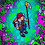## Sunday, June 11, 2023

### TibiaGoals Riddles E30R03

Comment down below!
Please keep track of the results HERE and inform us if there is any mistake via Discord, e-mail or Instagram.

1.Meregirl

https://imgur.com/a/NvGFmQW

2.Lyh

https://imgur.com/a/SgBiuF3

3.Presckot

6x6=36
Remove the right-top arrow of the first 8

4.1 from 8 to make 6, 6x6 = 36

5.6.remover one arrow to east of number 8 for equation 6x6=36
Baby Valen

7.draw an arrow from the number eight ... equation is 6x6=36
Baby Karol

8.6x6=36

Daso II

9.Remove the top right arrow on the 8 to create 6x6=36 - Libi

10.Character: Mikhelle
Answer: remove 1 arrow on the 8 to make it a 6 (top-right arrow)

11.Llama Llama - turn the 8 into a 6 by removing the top right arrow. 6x6=36

12.Remove the top right arrow on the 8 to create 6x6=36 tilo khalex

13.Remove the top right arrow on the 8 to create 6x6=36

Eveh

14.Remove the upper right vertical arrow of the number 8 so that it forms a 6 instead. 6 x 6 = 36.

-Essiee

15.6x6=36

16.Character: Arcai
6X6=36

17.Char: Zari Alnia

18.On the 8 remkve top right arroe to turn it into a 6. 6x6=36

19.Top right arrow on the 8 to turn it into a 6. 6x6=36
Esus Ang Warman

20.Gzew
Remove arrow on 8 to make a 6. So 6x6=36

21.Character: Tiger Backwoods
6 x 6 = 36

22.Turn the 8 into 6 to make it 6 x 6 = 36

Character Name: Dyaniixz

23.Turn the 8 into 6 to make it 6 x 6 = 36

Character Name: Dyaniixz

24.Turn the 8 into 6 to make it 6 x 6 = 36

Character Name: Dyaniixz

25.I must remove an arrow from the number 8 to convert it into 6, leaving the equation 6*6=36

26.remove one arrow to make 8 into 6

Daniellee

27.remove arrow from number 8 to make it a 6
6x6 is 36
character name: pablo reformed

28.remove arrow from number 8 to make it a 6
6x6 is 36
character name: pablo reformed

29.https://i.imgur.com/JK5Tn4y.png

bomdrax (yonabra)

30.Hymura: turn the 8 into a 6 to make the equation correct. 6x6=36

31.32.33.Smucker

6x6=36

34.Name Char: Hinata Hyuga Byakugan

Respuesta: 6x6 = 36

35.Name Char: Kathryna
Respuesta: 6x6 = 36

36.6x6=36
Braff herzs

37.Nick: Eriica Sar

By removing top right arrow on first number 8 to obtain a number 6, 6x6=36

38.Remove the arrow on number 8 to make it a 6 to get 6x6= 36

39.Remove the arrow on number 8 to make it a 6 to get 6x6=36

Juankz

40.Respuesta: 6x6 = 36

41.Name Char: Odrarcus Niel
6x6 = 36

42.Hi!
Remove the to right arrow on the number 8 to make 6x6 = 36
Cinderella Sea

43.Name Char: Yauraill Maleld

Respuesta: 6x6=36

44.Psykeeh

6x6 = 36

45.Ozud

Remove 1 arrow from 8 to make it a 6

46.Name Char: Thardorine Zhan

6x6=36

47.Chart: Alhejandra
Respuesta: 6 x 6 = 36

48.name: Neutrozx
Respuesta: la ecuación es 6 x 6 = 36

49.6x6=36 remove top right arrow on the 8. Tilo Khalex

50.chart name: Chaviitoh
Respuesta: 6 x 6 = 36

51.Remove the top right arrow turning the 8 into a 6. 6x6=36
Sosseguilda

52.Remove top right arrow from the 8 to make it a 6... 6x6 = 36

- Napom

53.Name Chart: Kriszaks
Respuesta; 6 x 6 = 36

54.Name: Korynita
Respuesta: 6 x 6 = 36

55.Remove the top arrow on the right side from number 8 to make it a 6
6x6 = 36

Sky Elie <3

56.6x6=36

Righo

57.PAN CETA

6 X 6 = 36

58.6 X 6 = 36

Lervan

59.Remove the top right arrow on the 8 to create 6x6=36

Ramona Toledo

60.Kriizs

6x6 = 36

61.Character: Glex Lobera

R: 6 x 6 = 36

62.R= 6 x 6 = 36
Samuray de carro :)

63.R= 6 x 6 = 36
Samuray de carro :)

64.R= 6 x 6 = 36
Samuray de carro :)

65.Remove one arrow from the 8 to make it a 6 so it ends up being 6x6=36
- Babygirrl

66.6 x 6 = 36 (Removing one arrow from the 8)
Green Khepri

67.6x6=36
Soma deux

68.6x6 = 36

Amy Meow

69.6x6=36
Hefrus

70.6 x 6 = 36

Char: Mia Moonshine

71.6 x 6 = 36

72.73.Just need to remove one arrow from the top of the 8 to make it 6 and should look like 6x6=36
-Sapo Dungeon

74.6x6=36 (remove an arrow from 8)
-Tanabata

75.remove an arrow from the 8 (should end as 6x6=36)
-Bara de Pan

76.6x6=36, Sir coor

77.Remove the upper right-hand side arrow from the "8" so it becomes a "6".
- Hunter of Dragoes

78.Change first 8 by 6 - Arabella Drummond

79.6 x 6 = 36 (Removing one arrow from the 8 than its a 6)
Vujataxu